# Spot the place where the file is dynamically loaded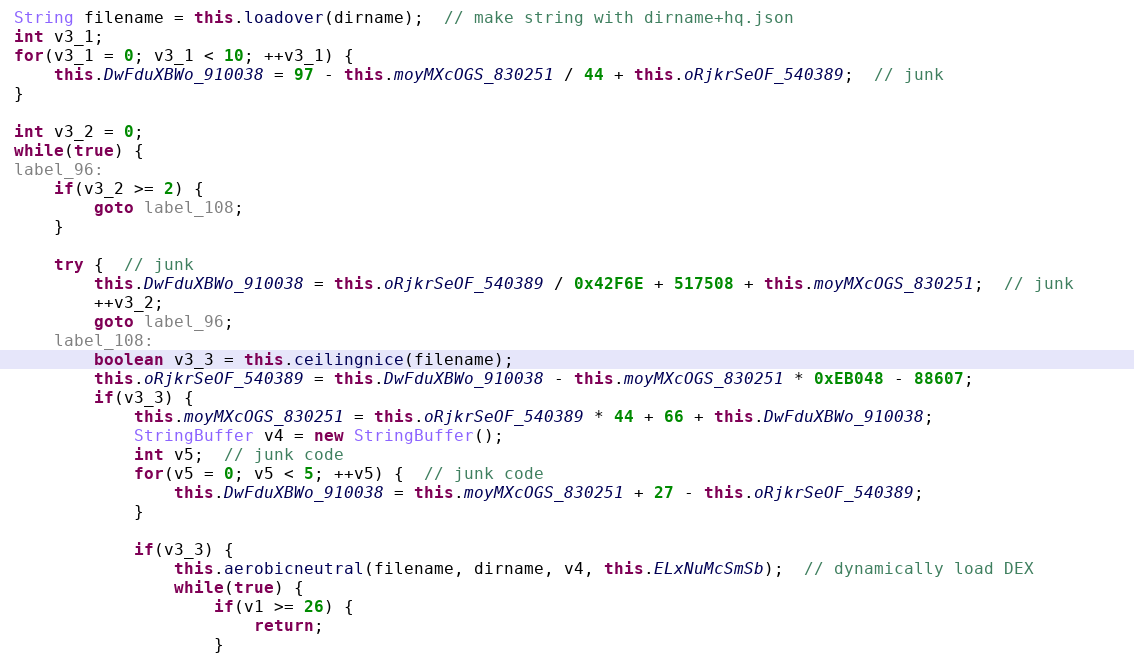Parts of code of attachBaseContext. There is lots of junk code. The payload filename is used 3 times in this screenshots: loadover, ceilingnice, aerobicneutral

# Spot where payload decryption occurs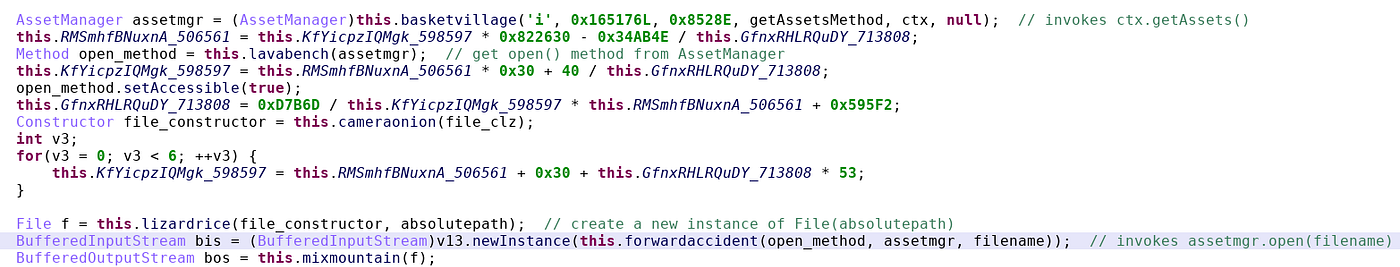First, the code retrieves an AssetManager. Then it opens the encrypted payload asset. The input stream is the encrypted payload, the output stream will be stored in the location of absolutepath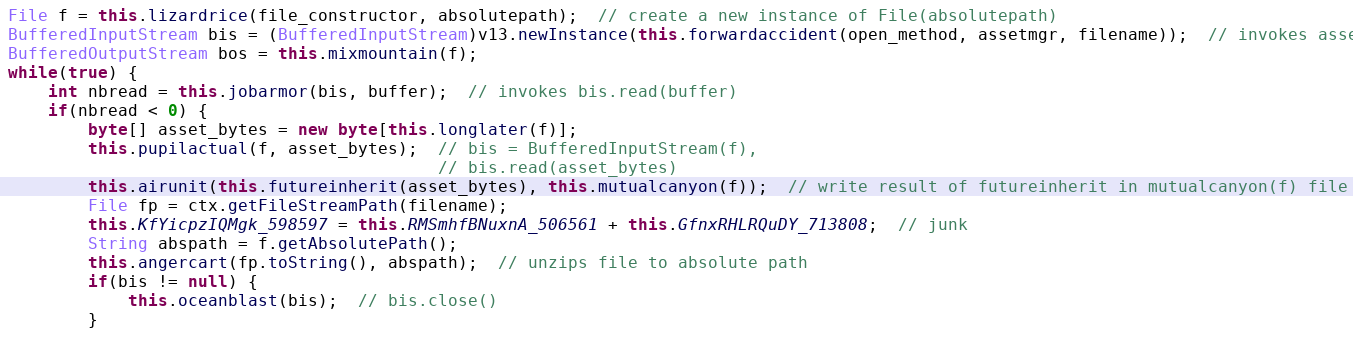This part of code (inside orcharddecide) decrypts the assets and unzips the result.

# Understanding the preparation of the key

`int cv = (int)StrictMath.hypot(this.timeone('d', 0x1E681L, convertedkey, index), 0.0);`
`private void swap(int a, int b, int[] array) {        int tmp = array[a];        array[a]=array[b];        array[b]=tmp;}    private int[] convert_key(byte[] key) {        int[] convertedkey = new int[0x100];        int i;        for(i = 0; i < 256; ++i) {            convertedkey[i] = i;  // init        }        int j = 0;        int k = 0;        while(j < 0x100) {            int cv = convertedkey[j];            k = (k+cv+key[j%key.length]+0x100) %  0x100;            swap(j, k, convertedkey);  // swap values            ++j;        }        return convertedkey;}`

# Decryption algorithm

`decrypted[i] = this.motionavoid(Math.round(v0_6) ^ encrypted[i]);`
`int v15 = this.timeone('b', 5222L, ckey, HMoEsEkXySsLhTyCkZlChSoBfFlPk.counter);  // ckey[counter]this.GfnxRHLRQuDY_713808 = this.KfYicpzIQMgk_598597 * 0x12FC3 + this.RMSmhfBNuxnA_506561 - 50009; // junkint v0_5 = this.timeone('z', 0x179161L, ckey, v14);  // ckey[v14]`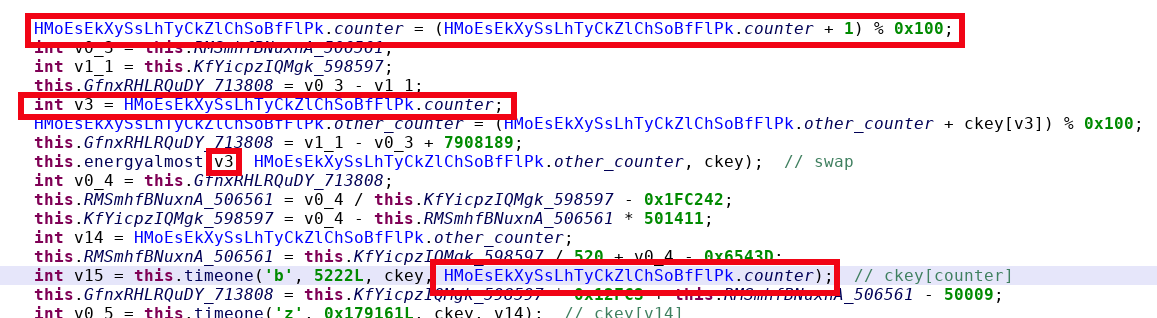The first use basically increments the counter, making sure it remains below 0x100. Then, counter is put in v2 and swapped with another value (energyalmost is a method that performs byte swap). Finally, v15 gets the value of the ckey[counter]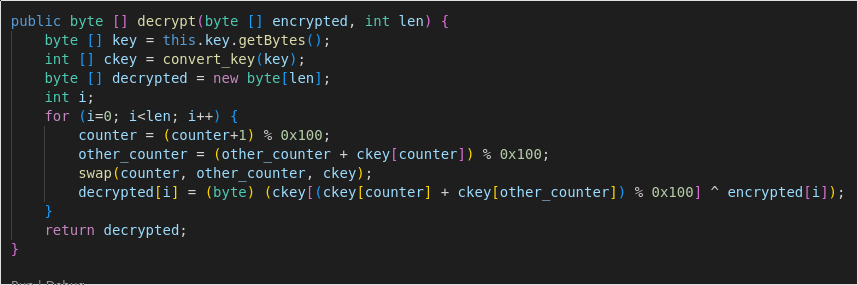Simplified decryption method. For this sample, the initial key is “Ianj”. The encrypted byte array is the contents of hq.json. I added a length argument because actually in my code the hq.json is read into a bigger array, and we only need to decrypt up to the length of hq.json file.